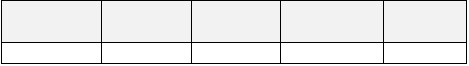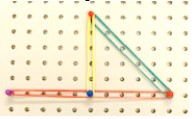# MA.8.GR.1.3Export Print
Use the Triangle Inequality Theorem to determine if a triangle can be formed from a given set of sides. Use the converse of the Pythagorean Theorem to determine if a right triangle can be formed from a given set of sides.
General Information
Subject Area: Mathematics (B.E.S.T.)
Strand: Geometric Reasoning
Status: State Board Approved

## Benchmark Instructional Guide

### Terms from the K-12 Glossary

• Converse of the Pythagorean Theorem

### Vertical Alignment

Previous Benchmarks

Next Benchmarks

### Purpose and Instructional Strategies

In grade 5, students classified triangles based on their angle measures and their side lengths. In grade 8, students use the Triangle Inequality Theorem and Pythagorean Theorem to determine whether triangles, or right triangles, can be formed from a given set of sides. In Geometry, students will extend this understanding to prove relationships and theorems about triangles.
• Instruction includes modeling and drawing triangles with different side lengths to determine if they can make a triangle to help in conceptual understanding. Students can physically construct triangles with manipulatives such as straws, sticks, string or geometry apps prior to using rulers (MTR.2.1).
• Exploration should involve giving students three side measures to determine if a triangle can be made. Through discussion of their exploration results, students should conclude that triangles cannot be formed by any three arbitrary side measures.
• For example, if students are given 4, 5 and 10, they should conclude that it does not form a triangle.
• Through charting, students should realize that for a triangle to result, the sum of any two side lengths must be greater than the third side length. This can be charted in a table like the one below.• Once students understand the Triangle Inequality Theorem, they can apply their knowledge to the converse of the Pythagorean Theorem. In work with the previous benchmark, students verify using a model that the sum of the squares of the legs is equal to the square of the hypotenuse in a right triangle. Students should also understand that if the sum of the squares of the 2 smaller legs of a triangle is equal to the square of the third leg, then the triangle is a right triangle.

### Common Misconceptions or Errors

• Students may incorrectly think that the Triangle Inequality Theorem only applies to right triangles due to the work with the Pythagorean Theorem. Discussion of the two theorems and examples will help with this misconception.
• Students may incorrectly believe endpoints of the sides of the triangle do not have to meet at a vertex.
• For example, students will attempt to make a triangle such as the example below.### Strategies to Support Tiered Instruction

• Instruction includes co-constructing a graphic organizer to highlight key differences and use of the Pythagorean Theorem and the Triangle Inequality Theorem.
• Instruction includes the use of geometric software to allow for students to explore the similarities and differences between the Pythagorean Theorem and the Triangle Inequality Theorem.
• Teacher provides instruction on the definition of a triangle and allows for students to explore various side lengths using geometric software or manipulatives to determine if the lengths form a triangle.

The following side lengths, in meters, were given to a carpenter to build a front porch with a triangular design. The carpenter needs to determine which set of lengths will make a triangle to be able to use it in his design.
Option 1: Side lengths: 4, 4, 8
Option 2: Side lengths: 6, 8, 10
Option 3: Side lengths: 6, 6, 13
• Part A. Which of the options would create a triangle for his design?
• Part B. The homeowner would like the porch to be in the shape of a right triangle. Will the carpenter be able to use any of the given options?
• Part C. For any option that does not form a triangle, what side length could be changed to form a triangle? Explain your answer.

### Instructional Items

Instructional Item 1
Can the side lengths of a triangle be 2, 4 and 8? Justify your answer.

Instructional Item 2
John drew a triangle with side lengths of 5, 12 and 13. His friend, Bryan, looked at it and asked John if it is a right triangle. John’s response was yes. Explain or show how John can prove to Bryan that the triangle is a right triangle.

*The strategies, tasks and items included in the B1G-M are examples and should not be considered comprehensive.

## Related Courses

This benchmark is part of these courses.
1205050: M/J Accelerated Mathematics Grade 7 (Specifically in versions: 2014 - 2015, 2015 - 2020, 2020 - 2022, 2022 and beyond (current))
1205070: M/J Grade 8 Pre-Algebra (Specifically in versions: 2014 - 2015, 2015 - 2022, 2022 and beyond (current))
1204000: M/J Foundational Skills in Mathematics 6-8 (Specifically in versions: 2014 - 2015, 2015 - 2022, 2022 and beyond (current))
7812030: Access M/J Grade 8 Pre-Algebra (Specifically in versions: 2014 - 2015, 2015 - 2018, 2018 - 2019, 2019 - 2022, 2022 and beyond (current))

## Related Access Points

Alternate version of this benchmark for students with significant cognitive disabilities.
MA.8.GR.1.AP.3a: Measure the sides of triangles to establish facts about the Triangle Inequality Theorem (i.e., the sum of two side lengths is greater than the third side).
MA.8.GR.1.AP.3b: Substitute the side lengths of a given figure into the Pythagorean Theorem to determine if a right triangle can be formed.

## Related Resources

Vetted resources educators can use to teach the concepts and skills in this benchmark.

## Formative Assessments

Sides of Triangles:

Students are asked to determine if given lengths will determine a triangle.

Type: Formative Assessment

Drawing Triangles SSS:

Students are asked to draw a triangle with given side lengths, and explain if these conditions determine a unique triangle.

Type: Formative Assessment

## Original Student Tutorial

Pythagorean Theorem: Part 1:

Learn what the Pythagorean Theorem and its converse mean, and what Pythagorean Triples are in this interactive tutorial.

This is part 1 in a 3-part series. Click below to explore the other tutorials in the series.

Type: Original Student Tutorial

## Tutorials

Construct a Right Isosceles Triangle:

This video discusses constructing a right isosceles triangle with given constraints and deciding if the triangle is unique.

Type: Tutorial

Construct a Triangle with Given Side Lengths:

This video demonstrates drawing a triangle when the side lengths are given.

Type: Tutorial

## MFAS Formative Assessments

Drawing Triangles SSS:

Students are asked to draw a triangle with given side lengths, and explain if these conditions determine a unique triangle.

Sides of Triangles:

Students are asked to determine if given lengths will determine a triangle.

## Original Student Tutorials Mathematics - Grades 6-8

Pythagorean Theorem: Part 1:

Learn what the Pythagorean Theorem and its converse mean, and what Pythagorean Triples are in this interactive tutorial.

This is part 1 in a 3-part series. Click below to explore the other tutorials in the series.

## Student Resources

Vetted resources students can use to learn the concepts and skills in this benchmark.

## Original Student Tutorial

Pythagorean Theorem: Part 1:

Learn what the Pythagorean Theorem and its converse mean, and what Pythagorean Triples are in this interactive tutorial.

This is part 1 in a 3-part series. Click below to explore the other tutorials in the series.

Type: Original Student Tutorial

## Tutorials

Construct a Right Isosceles Triangle:

This video discusses constructing a right isosceles triangle with given constraints and deciding if the triangle is unique.

Type: Tutorial

Construct a Triangle with Given Side Lengths:

This video demonstrates drawing a triangle when the side lengths are given.

Type: Tutorial

## Parent Resources

Vetted resources caregivers can use to help students learn the concepts and skills in this benchmark.